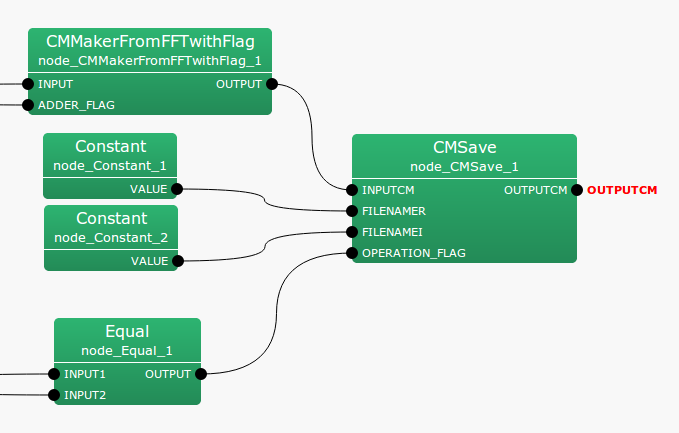## 6.2.2 CMSave

### 6.2.2.1 Module Overview

Saves the sound source correlation matrix to a file.

None.

### 6.2.2.3 Usage

In what case is the node used?

Use when saving the correlation matrix for a sound source, created from CMMakerFromFFT  or CMMakerFromFFTwithFlag , etc.

Typical Examples

Figure. 6.16 shows a usage example of CMSave  node.

INPUTCM input terminal is connected with a correlation matrix calculated from CMMakerFromFFT  or CMMakerFromFFTwithFlag , etc.

• Before Version 2.0
The type is Matrix<complex<float> >  type, but to process correlation matrices, convert it from a three dimensional complex array to a two dimensional complex array and then output. FILENAMER and FILENAMEI are string  type inputs, each indicating the saved files name containing the real and imaginary parts of the correlation matrix.

• After Version 2.1
Saved as a zip file. Only FILENAMER is used, and FILENAMEI is ignored.

OPERATION_FLAG is int  type or bool  type input, specifying when the correlation matrix is read. (Figure. 6.16 shows the connection of an Equal node as an example. However, if the node can output int  type or bool  type, then it does not matter at all).Figure 6.16: Network Example using CMSave

### 6.2.2.4 I/O and property setting of the node

Table 6.17: Parameter list of CMSave
 Parameter Type Default Unit Description ENABLE_DEBUG false ON/OFF of debugging information output

Input

INPUTCM

: Matrix<complex<float> >  type. Correlation matrix of each frequency bin. $M$-th order complex square array with correlation matrix outputs $NFFT/2 + 1$ items. Matrix<complex<float> >  indicates the row of frequency ($NFFT/2 + 1$ rows), and column of complex correlation matrix ($M * M$ columns).

FILENAMER

: string  type. Name of file containing the real part of the correlation matrix.

FILENAMEI

: string  type. Name of file containing the imaginary part of the correlation matrix.

OPERATION_FLAG

: int  type or bool  type. Only when this input terminal is 1 or when true, the correlation matrix is saved.

Output

OUTPUTCM

: Matrix<complex<float> >  type. Same as INPUTCM.

Parameter

ENABLE_DEBUG

: bool  type. Default value is false. When true, it is output to the standard output when saving the correlation matrix.

### 6.2.2.5 Module Description

• Before Version 2.0
Corrects the correlation matrix to Matrix<float>  type to a $M$-th order complex square array for each frequency bin, and saves with the specified file name. Saved files are divided into real and imaginary parts of the correlation matrix. If the number of frequency bins is $k$, and ($k = NFFT/2 + 1$), then the saved file will store $k$ rows and $M^2$ columns each.

• After Version 2.1
Save a set of correlation matrices of a $M$-th order complex square array for each frequency bin as a zip file.

Saving is performed only when OPERATION_FLAG is 1 or true.